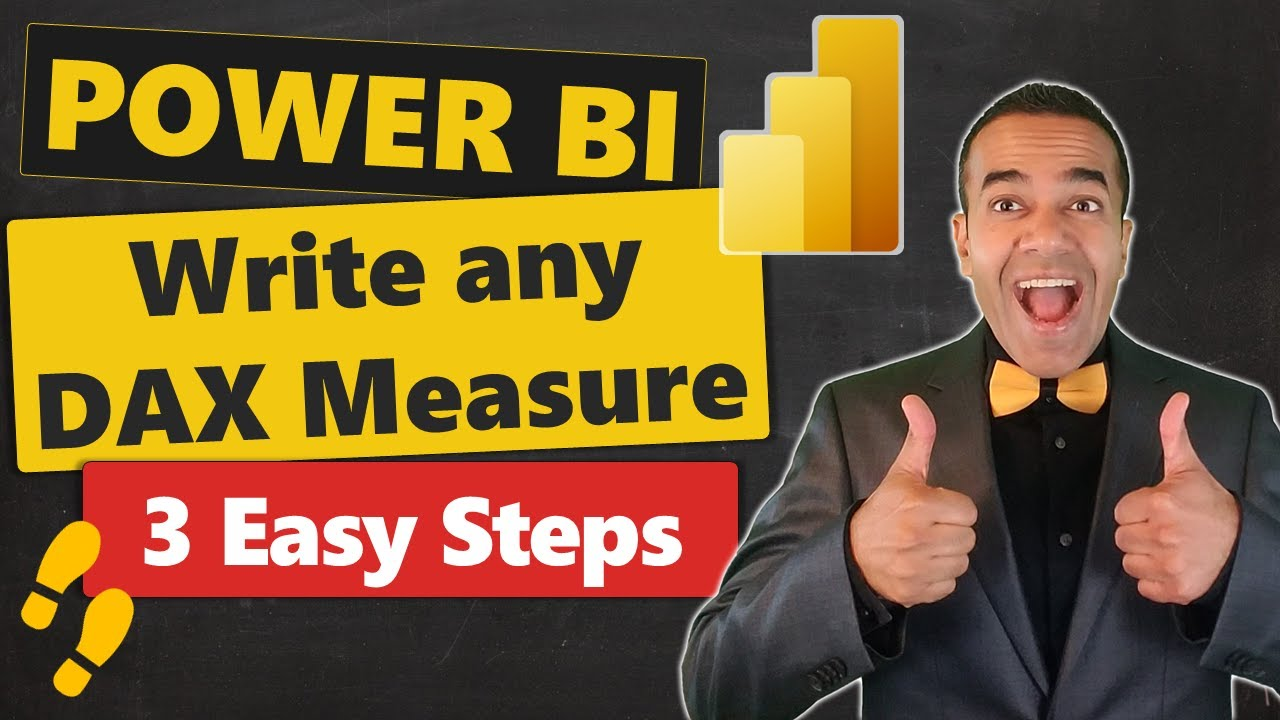en | deJun 30, 2023 1:00 PM

# Power BI: How to Write any DAX Measure In Just 3 Easy Steps

## Are you struggling to write DAX Measures? In this video I explain 3 Simple Steps that Non-Techie Business Users can take to write any DAX Measure.

Power BI is a powerful tool that utilizes Data Analysis Expressions (DAX) to perform custom calculations on data. These calculations can be simple sums or complex computations like year-on-year growth or average sales. The challenge with DAX lies in its complexity, making it difficult for Non-Techie Business Users. However, three easy steps can simplify this process: Human Learning, Talk Like a Baby, and Machine Learning.

• Step 1: Human Learning - absorbing all the necessary information about DAX.
• Step 2: Talk Like a Baby - breaking down the language of DAX into simpler terms.
• Step 3: Machine Learning - understanding how the system interprets and uses DAX.

Furthermore, understanding the CALCULATE function and being able to recap the steps provides mastery over Power BI. Evaluated in the context of the data model, a DAX measure performs calculations based on the current row or cell selection.

## Diving Deeper into Power BI and DAX

DAX in Power BI is a critical aspect for any individual working with data analysis services or Power Pivot in Excel. Its primary utility lies in its capability to perform custom calculations on data, creating calculated columns, tables or measures. For gaining expertise in DAX measures, one must have substantial knowledge of the language and system interpretation, besides being open to constant learning.

## Learn about Power BI: How to Write any DAX Measure In Just 3 Easy Steps

DAX (Data Analysis Expressions) is a library of functions used in Power BI, Analysis Services, and Power Pivot in Excel. It allows users to perform custom calculations on their data, such as creating calculated columns, calculated tables, or measures. A DAX measure is a formula that is evaluated in the context of the data model, taking the current row or cell selection into account when performing a calculation. Power BI users should learn about the various DAX functions available, how to write their own DAX measures, and the advantages of using DAX for data analysis. It is important to understand the basics of the CALCULATE function as well as the different types of DAX measures available. Additionally, users should understand the concept of context and how it affects the results of a measure. Finally, users should be familiar with the concept of human learning and machine learning in order to leverage the power of DAX. By following the 3 easy steps outlined in the video, users can understand and write any DAX measure.

### More links on about Power BI: How to Write any DAX Measure In Just 3 Easy Steps

Power BI DAX Tutorial for Beginners
Start by giving the formula a name and then follow with the equal-to sign (“=”). Then write in your formula using functions, constants, or strings.
Learn How to Write DAX in Power BI
Apr 19, 2023 — Measures and calculated columns both use DAX expressions. The difference is the context of evaluation. A measure is evaluated in the context of ...
All about the Power BI Calculate Function Simplified 101
Mar 3, 2022 — This article provides a comprehensive guide on Power BI CALCULATE Function. It also provides information on Power BI and DAX Functions.
A Complete Guide to Learn Power BI DAX
The following steps make your task simple to create a measure using Power BI DAX. 1. First, go to the Power BI Desktop app. Power BI DAX Desktop App. 2. Right- ...

### Keywords

1. DAX Measures 2. Power BI 3. CALCULATE Function 4. Year-over-Year Growth 5. Average Sales

HubSite 365 Apps